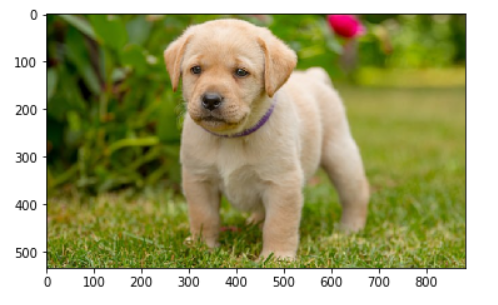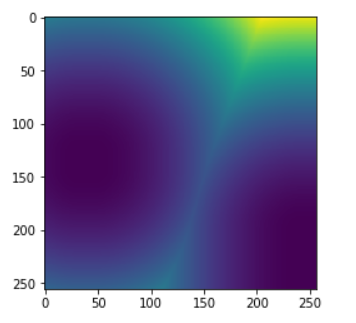# Python Mahotas – Introduction

Mahotas is a computer vision and image processing and manipulation library for Python. A library is a collection of functions and methods that allows you to perform many actions without having to write hundreds of lines of code. Mahotas includes many algorithms that operates with arrays, mahotas currently has over 100 functions for image processing and computer vision and is constantly growing.

Mahotas provides a good solution in finding patterns in the image for example “Where’s Wally Problem” can be solved easily using Mahotas.

How to install Mahotas :

`pip install mahotas`

Notable algorithms available in Mahotas :

1. Watershed
2. Convex points calculations.
3. Hit & miss, thinning.
4. Zernike & Haralick, LBP, and TAS features.
5. Speeded-Up Robust Features (SURF), a form of local features.
6. Thresholding.
7. Convolution.
8. Sobel edge detection.
9. Spline interpolation
10. SLIC super pixels.

 `# importing required libraries ` `import` `numpy as np ` `import` `mahotas ` `import` `pylab ` ` `  `# loading iamge ` `img ``=` `mahotas.imread(``'dog_image.png'``) ` ` `  `# showing the original image ` `imshow(img) ` `show() `

Output :Example 2: Creating distance transform

 `# importing required libraries ` `import` `pylab as p ` `import` `numpy as np ` `import` `mahotas ` ` `  `# creating numpy array of type bool ` `f ``=` `np.ones((``256``, ``256``), ``bool``) ` ` `  `# setting false values ` `f[``200``:, ``240``:] ``=` `False` `f[``128``:``144``, ``32``:``48``] ``=` `False` ` `  `# f is basically True with the exception of two islands: ` `# one in the lower-right ` `# corner, another, middle-left ` ` `  `# creating a distance using numpy array ` `dmap ``=` `mahotas.distance(f) ` ` `  `# showing iamge ` `p.imshow(dmap) ` `p.show() `

Output :My Personal Notes arrow_drop_upCheck out this Author's contributed articles.

If you like GeeksforGeeks and would like to contribute, you can also write an article using contribute.geeksforgeeks.org or mail your article to contribute@geeksforgeeks.org. See your article appearing on the GeeksforGeeks main page and help other Geeks.

Please Improve this article if you find anything incorrect by clicking on the "Improve Article" button below.

Article Tags :

1

Please write to us at contribute@geeksforgeeks.org to report any issue with the above content.